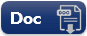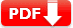# Pre-Algebra Practice Test Question Answers [Test SET 2]

[Test SET 2]

 1. If 3x = y – 5 and x = -4, then y =? 🔘 -7 🔘 5 🔘 9 🔘 -9 🔘 7
 2. If 9 is P percent of 17, then P is a number between 🔘 10 to 50 🔘 20 to 40 🔘 30 to 60 🔘 20 to 60 🔘 40 and 60
 3. 2.5 ÷ 1000 is equal to 🔘 0.025 🔘 0.0025 🔘 0.2500 🔘 25000 🔘 0.250
 4. \sqrt{6^2+3^2} 🔘7.2 🔘 7.9 🔘 13.6 🔘 14.6 🔘 17.0
 5. A basketball team won 10 games and lost 15. What is the ratio of wins to the total number of games? 🔘 \frac32 🔘 \frac23 🔘 \frac27 🔘 \frac37 🔘 NOTA
 6. Which of the following is the prime factorization of 28? 🔘 2x2x7 🔘 2×7 🔘 2x3x7 🔘2x3x3 🔘 2x5x7
 7. 4(x + 6) + 2(3x – 5) =? 🔘 2x2x7 🔘 2×7 🔘 2x3x7 🔘2x3x3 🔘 2x5x7
 8. The area A of a circle is given by the formula: A = πr2 If the radius of the circle is 7 inches, find the area of the circle. 🔘 7π sq. inches 🔘 28π sq. inches 🔘 39π sq. inches 🔘41π sq. inches 🔘 49π sq. inches
 Document Type Download Link Free Editable Doc FileFree Printable PDF File